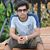# B.TECH 7th sem Electrical Machine Design paper 2017

UniversityGGSIPU, New Delhi
CourseB.TECH
Semester7
SubjectElectrical Machine Design
Year2017
Downloads6
Uploaded By###### Sourabh Bajaj
200

 Question Marks 1 requests (a) State the factors which affect the choice of ampere conductor per meter of a DC machine. This question has 0 answers so far. 6 1 requests (b) What are the guiding factors for choice of number of poles in a DC machine?.  This question has 0 answers so far. 6.5 1 requests (a) Describe the factors which should be considered when selecting the core length are armature diameter of a DC machine?  This question has 0 answers so far. 6 1 requests (b) Determine the main dimensions of a 5 kW, 250 V, 4-pole, 1500 rpm DC shunt generator having a square pole face. Assume the average flux density in air gap = 0.42 We/m2, ampere conductors per meter = 15000, full load efficiency = 87% and ratio of pole arc to pole pitch = 0.66. This question has 0 answers so far. 6.5 1 requests (a) Describe the different methods for cooling the dry and oil immersed types of transformes.  This question has 0 answers so far. 6.5 1 requests (b) What is the window space factor of a transformer? Derive the expressions for the window dimensions of a transformer. This question has 0 answers so far. 6 1 requests (a) Derive the expression for voltage per turn for a transformer. This question has 0 answers so far. 5.5 1 requests (b) Determine the dimensions of core and yoke for a 200 kVA, 50 Hz core type single phase transformer. A cruciform core is used with distance between adjacent limbs equal to 1.6 times the width of core laminations. Assume voltage per turn 14 V, maximum flux density 1.1 Wb/m2, current density 3 A/mm2, window space factor 0.32 and stacking factor = 0.9. The net iron area is 0.56 d2 in a cruciform core where d is the diameter of circumscribing circle. Also the width of largest stamping is 0.85 d.  This question has 0 answers so far. 7 1 requests (a) What are the guiding factors for selecting the rotor slots of a squirrel cage induction motor? This question has 0 answers so far. 6 1 requests (a) What are the guiding factors for selecting the rotor slots of a squirrel cage induction motor? This question has 0 answers so far. 6 1 requests (b) Describe the methods used for reduction of harmonic torques in an induction motor.  This question has 0 answers so far. 6.5 1 requests Determine the main dimensions, turns per phase, total conductors and number of slots of a 250 H.P., 3-phase 50 Hz, 400 V, 1410 rpm slip ring induction motor. Assume Bay = 0.5 Wb/m2, ac = 3000 A/m, efficiency = 90%, power factor = 0.9, winding factor = 0.955, current density = 3.5 A/mm2. The slot space factor is 0.4 and the ratio of core length to pole pitch is 1.2. The machine is delta connected. This question has 0 answers so far. 12.5 1 requests (a) Describe the factors that influence the choice of average flux density in designing the synchronous machines.  This question has 0 answers so far. 6.5 1 requests (b) Illustrate the methods used for selecting the diameter of stator bore for designing a salient pole synchronous machine. This question has 0 answers so far. 6 1 requests (a) Revolving field and stationary armature in synchronous machine.  This question has 0 answers so far. 6.5 0 requests (b) Damper winding in synchronous machines. This question has 0 answers so far. 6 0 requests Write the precise and complete answer of each of the following:(a) Define two types of specific loadings used in designing of rotating electrical machines and state how they affect the size of the machines. This question has 0 answers so far. 5 0 requests Write the precise and complete answer of each of the following:(b) What are the main dimensions of a DC machine? Derive the outpute quation of a DC machine in terms of its main dimensions. This question has 0 answers so far. 5 0 requests Write the precise and complete answer of each of the following:(c) Write the conditions to design a transformer for minimum total cost,minimum volume, minimum weight and minimum losses. This question has 0 answers so far. 5 0 requests Write the precise and complete answer of each of the following:(d) State how the power factor and the magnetizing current of an induction motor are affected by the length of its air gap. This question has 0 answers so far. 5 0 requests Write the precise and complete answer of each of the following:(e) How does the short circuit ratio (SCR) of a synchronous machine affect its performance?  This question has 0 answers so far. 5
Chat with us